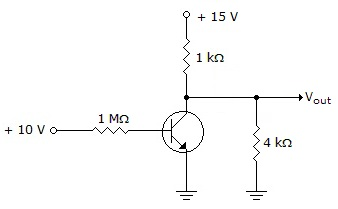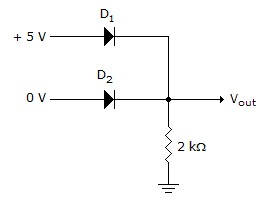# Digital Electronics - Signals and Switches

### Exercise :: Signals and Switches - General Questions

11.

How long will it take to serially transmit the hexadecimal number C316 if the clock frequency is 1.5 MHz?

 A. 4.69 ms B. 4.69s C. 5.33 ms D. 5.33s

Answer: Option D

Explanation:

No answer description available for this question. Let us discuss.

12.

What is the output voltage from the circuit in the given figure?A. +15 V B. +12 V C. +3 V D. 0 V

Answer: Option D

Explanation:

No answer description available for this question. Let us discuss.

13.

The family of logic that is composed of bipolar junction transistors is ____.

 A. TTL B. CMOS C. DIP D. BJT

Answer: Option A

Explanation:

No answer description available for this question. Let us discuss.

14.

What can a relay provide between the triggering source and the output that semiconductor switching devices cannot?

 A. total isolation B. faster C. higher current rating D. total isolation and higher current rating

Answer: Option D

Explanation:

No answer description available for this question. Let us discuss.

15.

Determine the output voltage Vout for the circuit in the given figure.A. –0.5 V B. 0 V C. +5 V D. +4.3 V

Answer: Option D

Explanation:

No answer description available for this question. Let us discuss.

#### Current Affairs 2021

Interview Questions and Answers